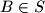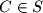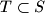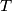### IMO Shortlist 2008 problem C3

Kvaliteta:
Avg: 0,0
Težina:
Avg: 7,0
In the coordinate plane consider the set$S$ of all points with integer coordinates. For a positive integer$k$, two distinct points$a$,$B\in S$ will be called$k$-friends if there is a point$C\in S$ such that the area of the triangle$ABC$ is equal to$k$. A set$T\subset S$ will be called$k$-clique if every two points in$T$ are$k$-friends. Find the least positive integer$k$ for which there exits a$k$-clique with more than 200 elements.

Proposed by Jorge Tipe, Peru
Izvor: Međunarodna matematička olimpijada, shortlist 2008